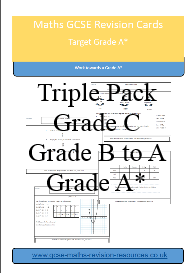Refer a friend and get % off! They'll get % off too.GCSE Maths Revision Cards Triple Pack Grade C, Grade B to A and Grade A*

GCSE Maths Revision Cards.

This is a triple pack containing the Grade C, Grade B to A and the Grade A Star Packs. Save by buying three packs together.

For further information on the contents of the packs or to preview the cards please follow the links on the website

This pack contains nearly 200 revision cards.

Pack Contents

Estimating the mean from grouped data
3D Coordinates
Add and Subtract Mixed Numbers
Addition and Subtraction of Fractions
Angles 1
Angles in a polygon
Angles in polygons 2
Angles on parallel lines
Area 1
Area 2
Area of a circle
Bearings
Best Value
Bounds
Circumference of a circle
Collecting Like terms.
Compound Area
Compound Measures
Conversion Graphs
Enlargement
Equations of Simple Lines
Equivalent Fractions
Expanding Single Brackets
Factorising Single Brackets
Find the mean, median, mode and range
Finding the mean, mode and range from a table
Forming and solving equations
Fraction of a Quantity
Frequency Polygons
Highest Common Factor
Indices
Inequalities
Linear Graphs
Long Multiplication
Long Multiplication with Decimals
Lowest Common Multiple
Metric / Imperial Conversions
Metric Conversions
Mixed Numbers and Improper Fractions
Money Conversions
Multiplication and Division of Fractions
Multiply and Divide Mixed Numbers
Multiplying and Dividing by a Power of 10
nth Term
Percentage Decrease
Percentage Increase
Percentage of a Number
Plans and elevations
Probability—expected outcomes
Product of Prime Numbers
Pythagoras’ Theorem
Questionnaires
Ratio
Ratio 2
Reflection
Rotation
Rounding—Decimal Places
Rounding—Significant Figures
Scatter Graphs
Solving Linear Equations
Stem and Leaf Chart
Substitution into Expressions
Sum of Probabilities
Surface Area
Tessellation
Translation
Trial and Improvement
Two Way Table from a Worded Question
Two Way Tables
Volume

Grade B to A pack topics

Grade B to A
Angle Bisector
Arc Length
Area of a Sector
Box Plot from a Cumulative Frequency Graph
Box Plot from Discrete Data
Calculations with Bounds
Circle Theorems 1
Circle Theorems 2
Circle Theorems 3
Circle Theorems 4
Comparing Box Plots
Compound Interest
Construct Triangles with Compasses
Constructing Perpendicular Lines
Convert a Recurring Decimal to a Fraction
Converting Numbers to Standard Form
Converting Square and Cube Units
Converting Standard Form to Numbers
Cumulative Frequency Graph
Cumulative Frequency Graph - Solving Problems
Depreciation
Direct Proportion
Draw a 30 Degree Angle
Enlargement by a Fractional Scale Factor
Enlargement by a Negative Scale Factor
Enlargement Finding Side Lengths
Equation of a Straight Line
Equation of a Straight Line Parallel Lines
Equidistant From a Point and a Line
Expanding Double Brackets
Factorising Double Brackets
Graphing Inequalities
Indices Fraction and Negative
Indirect Proportion
Inverse Percentages
Loci Problem
Make x the Subject of an Equation
Matching Graphs
Median and Inter Quartile Range from a Cumulative Frequency Graph
Mid Point of a Line
Probability - Tree Diagrams
Simultaneous Equations 1
Simultaneous Equations 2
Simultaneous Equations 3
Solving Quadratics by Factorisation
Standard Form Addition and Subtraction
Standard Form Multiplication and Division
Stratified Sample
Travel Graphs
Trigonometry Finding a Side Length
Trigonometry Finding an Angle
Volume and Surface Area of a Cylinder

Grade A Star pack topics

A*
3D Pythagoras
3D Trigonometry
Algebraic Fractions
Algebraic proof
Area of a Segment
Area of a Triangle
Circle Theorems 5
Circles and linear equations
Completing the square
Conditional Probability
Conditional Probability 2
Congruence 1
Congruence 2
Difference of 2 Squares
Drawing a Histogram
Equation of a circle
Equation of a line from 2 coordinates
Equation of a perpendicular line
Estimate the Median From Grouped Data
Finding an Angle Sine and Cosine Rule 1
Finding an Angle Sine and Cosine Rule 2
Finding an Angle Sine and Cosine Rule 3
Form and Solve a Quadratic Equation
Form and Solve a Quadratic Equation 2
Form and Solve a Quadratic Equation 3
Histogram - Calculate Frequencies
Histogram Calculating Values
Indices Power to a Power
Quadratic and linear equations
Rationalise the Denominator
Side Length Sine and Cosine Rule 1
Side Length Sine and Cosine Rule 2
simplifying algebraic fractions
Solving Equations Using Volumes
Solving Exponential Equations
Surds
Transformations of Functions 1
Transformations of Functions 2
Transformations of Trig Graphs 1
Transformations of Trig Graphs 2
Transformations of Trig Graphs 3
Transformations of Trig Graphs 4
Trigonometric Value of any Angle 1
Trigonometric Value of any Angle 2
Trigonometric Value of any Angle 3
Vectors
Vectors 2

You will get the following files:

• PDF (5MB)
• PDF (5MB)
• PDF (3MB)

£ 3.50

£ 3.50

Discount has been applied.

orAdding ...